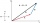# Add vector

Given that P = (5, 8) and Q = (6, 9), find the component form and magnitude of vector PQ.

Result

x =  1
y =  1
|PQ| =  1.41

#### Solution:Leave us a comment of example and its solution (i.e. if it is still somewhat unclear...):

Showing 0 comments:Be the first to comment!#### To solve this example are needed these knowledge from mathematics:

Two vectors given by its magnitudes and by included angle can be added by our vector sum calculator. Pythagorean theorem is the base for the right triangle calculator.

## Next similar examples:

1. Vector 7Given vector OA(12,16) and vector OB(4,1). Find vector AB and vector |A|.
2. Coordinates of vectorDetermine the coordinate of a vector u=CD if C(19;-7) and D(-16;-5)
3. AS sequenceIn an arithmetic sequence is given the difference d = -3 and a71 = 455. a) Determine the value of a62 b) Determine the sum of 71 members.
4. SeatsSeats in the sport hall are organized so that each subsequent row has five more seats. First has 10 seats. How many seats are: a) in the eighth row b) in the eighteenth row
5. Median and modusRadka made 50 throws with a dice. The table saw fit individual dice's wall frequency: Wall Number: 1 2 3 4 5 6 frequency: 8 7 5 11 6 13 Calculate the modus and median of the wall numbers that Radka fell.
6. 75th percentile (quartille Q3)Find 75th percentile for 30,42,42,46,46,46,50,50,54
7. Six termsFind the first six terms of the sequence a1 = -3, an = 2 * an-1
8. Quadratic equationFind the roots of the quadratic equation: 3x2-4x + (-4) = 0.
9. TetrahedronCalculate height and volume of a regular tetrahedron whose edge has a length 18 cm.
10. Euclidean distanceCalculate the Euclidean distance between shops A, B and C, where: A 45 0.05 B 60 0.05 C 52 0.09 Wherein the first figure is the weight in grams of bread and second figure is price in USD.
11. Theorem proveWe want to prove the sentense: If the natural number n is divisible by six, then n is divisible by three. From what assumption we started?
12. DiscriminantDetermine the discriminant of the equation: ?
13. EquationEquation ? has one root x1 = 8. Determine the coefficient b and the second root x2.
14. CalculationHow much is sum of square root of six and the square root of 225?
15. Sequence 3Write the first 5 members of an arithmetic sequence: a4=-35, a11=-105.
16. TrinityHow many different triads can be selected from the group 43 students?
17. Linsys2Solve two equations with two unknowns: 400x+120y=147.2 350x+200y=144